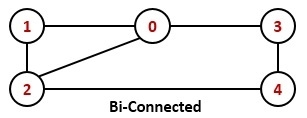# Biconnected Graph

Data StructureAlgorithmsGraph Algorithms

#### Big Data Hadoop

Best Seller

89 Lectures 11.5 hours

#### Practical Data Science using Python

22 Lectures 6 hours

#### Data Science and Data Analysis with Python

50 Lectures 3.5 hours

An undirected graph is said to be a biconnected graph, if there are two vertex-disjoint paths between any two vertices are present. In other words, we can say that there is a cycle between any two vertices.We can say that a graph G is a bi-connected graph if it is connected, and there are no articulation points or cut vertex are present in the graph.

To solve this problem, we will use the DFS traversal. Using DFS, we will try to find if there is any articulation point is present or not. We also check whether all vertices are visited by the DFS or not, if not we can say that the graph is not connected.

## Input and Output

Input:
The adjacency matrix of the graph.
0 1 1 1 0
1 0 1 0 0
1 1 0 0 1
1 0 0 0 1
0 0 1 1 0

Output:
The Graph is a biconnected graph.

## Algorithm

isArticulation(start, visited, disc, low, parent)

Input: The start vertex, the visited array to mark when a node is visited, the disc will hold the discovery time of the vertex, and low will hold information about subtrees. The parent will hold the parent of the current vertex.

Output − True if any articulation point is found.

Begin
time := 0      //the value of time will not be initialized for next function calls
dfsChild := 0
mark start as visited
set disc[start] := time+1 and low[start] := time + 1
time := time + 1

for all vertex v in the graph G, do
if there is an edge between (start, v), then
if v is visited, then
increase dfsChild
parent[v] := start

if isArticulation(v, visited, disc, low, parent) is true, then
return ture
low[start] := minimum of low[start] and low[v]

if parent[start] is φ AND dfsChild > 1, then
return true

if parent[start] is φ AND low[v] >= disc[start], then
return true
else if v is not the parent of start, then
low[start] := minimum of low[start] and disc[v]
done
return false
End

isBiconnected(graph)

Input: The given graph.

Output − True if the graph is bi-connected.

Begin
initially set all vertices are unvisited and parent of each vertices are φ
if isArticulation(0, visited, disc, low, parent) = true, then
return false

for each node i of the graph, do
if i is not visited, then
return false
done
return true
End

## Example

#include<iostream>
#define NODE 5
using namespace std;

int graph[NODE][NODE] = {
{0, 1, 1, 1, 0},
{1, 0, 1, 0, 0},
{1, 1, 0, 0, 0},
{1, 0, 0, 0, 1},
{0, 0, 0, 1, 0}
};

int min(int a, int b) {
return (a<b)?a:b;
}

bool isArticulation(int start, bool visited[], int disc[], int low[], int parent[]) {
static int time = 0;
int dfsChild = 0;
visited[start] = true;    //make the first vertex is visited
disc[start] = low[start] = ++time;    //initialize discovery time and the low time

for(int v = 0; v<NODE; v++) {
if(graph[start][v]) {   //for all vertex v, which is connected with start
if(!visited[v]) {
dfsChild++;
parent[v] = start;    //make start node as parent
if(isArticulation(v, visited, disc, low, parent))
return true;
low[start] = min(low[start], low[v]);    //when subtree from v is connected to one of parent of start node
if(parent[start] == -1 && dfsChild > 1) {    //when u have 2 or more children
return true;
}

if(parent[start] != -1 && low[v]>= disc[start])
return true;
} else if(v != parent[start])    //update low of start for previous call
low[start] = min(low[start], disc[v]);
}
}
return false;
}

bool isBiConnected() {
bool *vis = new bool[NODE];
int *disc = new int[NODE];
int *low = new int[NODE];
int *parent = new int[NODE];

for(int i = 0; i<NODE; i++) {
vis[i] = false;    //no node is visited
parent[i] = -1;    //initially there is no parent for any node
}

if(isArticulation(0, vis, disc, low, parent))    //when no articulation point is found
return false;
for(int i = 0; i<NODE; i++)
if(!vis[i])    //if any node is unvisited, the graph is not connected
return false;
return true;
}

int main() {
if(isBiConnected())
cout << "The Graph is a biconnected graph.";
else
cout << "The Graph is not a biconnected graph.";
}

## Output

The Graph is a biconnected graph.